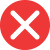20th

Correct spelling, explanation: this form is the correct one because the full-word form twentieth ends with th, thus this ending functions in the number form. For the same reason the suffix st is reserved for the ordinal number first (and any other number ending with first. Therefore 20th is correct and 20st is a mistake.

Definition of 20th:
numeral, the ordinal number between 19th and 21st
This is the 20th book he has written. I think this one is the best.
I want to travel to Rome on my 20th birthday.20st

Incorrect spelling, explanation: the correct form here should be 20th, not 20st, since the word twentieth ends with th, which means the number form should also end with th. St is the ending for the number first, and there is no first in twentieth. Therefore 20th is the correct form here.20nd

Incorrect spelling20rd

Incorrect spelling